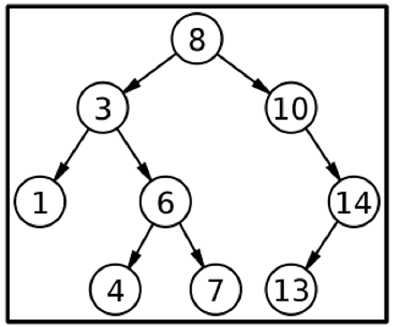# Finding the second largest element in BST using C++

In a binary search tree (BST), the second largest element must be returned. In a binary tree, the second element is the largest element.According to the given BST, 13 is the second largest element. Now we are using the C++ approach to solve this problem. We can traverse the tree inorder, and by observation, we can observe that the second largest element in the given BST is 13. The inorder of the tree will be 1 3 4 6 7 8 10 13 14, and we can observe that the elements are in the sorted array. So we return the second largest element.

Let’s assume some simple input and output scenarios

Assume the elements in a binary tree as shown in below. Here there are only two present in the binary search tree. By comparing the values of the nodes the second highest value wil be 6.

Input = BST
11
/
6
Output = Second largest element in BST will be: 6


Also consider this scenario below, as there is a binary search tree below and having good amount of nodes. The output of the below binary tree will be

Input = BST
11
/  \
6   21
/     \
4       31
Output = Second largest element in BST will be: 21

## Algorithm

The steps below is the following way to approach.

• Implement a Binary search tree (BST).

• Insert the values of the node into BST.

• Using inorder traversing technique, Left -> Root -> Right (L-R-R)

• Then find out the second largest node value in BST by using [inorder.size()-2];

## Example

Following is the C++ code to find the second largest element in a binary search tree −

#include <iostream>
#include <vector>
using namespace std;
class Node {
public:
int val;
Node *left, *right;
Node(int val) {
this->val = val;
left = right = NULL;
}
};
void solve(Node* root, vector<int>& inorder) {
if(root == NULL) return;
solve(root->left, inorder);
inorder.push_back(root->val);
solve(root->right, inorder);
}
int main() {
Node* root = new Node(8);
root->left = new Node(3);
root->right = new Node(10);
root->left->left = new Node(1);
root->left->right = new Node(6);
root->left->right->left = new Node(4);
root->left->right->right = new Node(7);
root->right->right = new Node(14);
root->right->right->left = new Node(13);
vector<int> inorder;
solve(root, inorder);
cout << "Second largest element of this BST is : " << inorder[inorder.size()- 2];
return 0;
}


## Output

Second largest element of this BST is: 13


## Conclusion

This is a purely observational-based question in which we need to observe the pattern with the inorder of the tree. The time complexity of this algorithm is O(n) for the inorder.

Updated on: 10-Aug-2022

420 Views